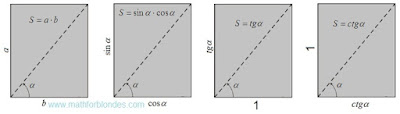## 8/11/2016

### Multiplication

Subject of occupations:
TRIGONOMETRIC FUNCTIONS IN A RECTANGLE
Subject of the previous lesson
Variable units of measure

Lesson 8

Multiplication

As a result of multiplication of two different quantities the third quantity turns out. At multiplication of change occurs both in a number domain, and in the field of units of measure. Multiplication is possible only for perpendicular quantities. Multiplication reflects high-quality changes of quantities.

Multiplication of the parties of a rectangle allows to receive the area.Multiplication of the parties of a rectangle

For transition from the area of a rectangle to the simple area it is necessary to divide the square into itself. In this case two options of algebraic transformations are possible. It is necessary to highlight that the recommendation of mathematicians about reduction of identical quantities in numerator and a denominator of fraction is consciously controlled for identification of quantity of the received result. The first option of transformations looks as follows.First option of the unit area

The tangent and cotangent are connected by the inverse proportion. The parties of a rectangle can change somehow, from infinitely big quantity to infinitesimal. If change of the parties is carried out with keeping of the inverse proportion, then the area of a rectangle will remain invariable.

The second option of transformations is presented in an algebraic form and in physical units of measure.Second option of the unit area

This transformation shows that as a result of multiplication of two different units the third unit turns out. Multiplication is an interaction of two perpendicular units of measure as a result of which the new unit of measure turns out. As any quantity is result of multiplication of number and a unit of measure, under figure "one" can the number, a unit of measure or result of their interaction is meant.

Perpendicular units of measure are not connected among themselves in any way and can have the arbitrariest scale. The scale of units of measure of factors scales work units of measure, but does not influence a process essence – multiplication leads to high-quality change of initial units of measure.

The area is a result of interaction of two perpendicular measurements of length. If to multiplication inches by meters or millimeters on meters, then the area will be expressed in inches on meter or millimeters on meter. Traditionally, the area can be expressed in identical units of measure of length and width. In practice frames with different scales on a vertical and a horizontal are often used.

At determination of the area of a square the size of the party can be built in the second degree. But it does not mean at all that the party of a square is multiplied by itself. The area can still be determined only by multiplication of length to width, just at a square they have identical numerical values. We never multiply rectangle length by length or width on width because the result of such actions does not make sense. The involution is a multiplication of the different perpendicular quantities having identical numerical values and units of measure.

Algebraic transformations of multiplication of two to a square can be written down so:Algebraic transformations

If to consider that quantity a at a quadrating is multiplied by itself, then the inverse transformation of a square in work of two sums will be impossible. As it is possible to execute a similitude, is shown in the partition Decomposition of sume below.

Diagonal of a rectangle and angle between the diagonal and the party are padding characteristics of the interacting quantities.

At the following lesson we will consider
Examples of multiplication